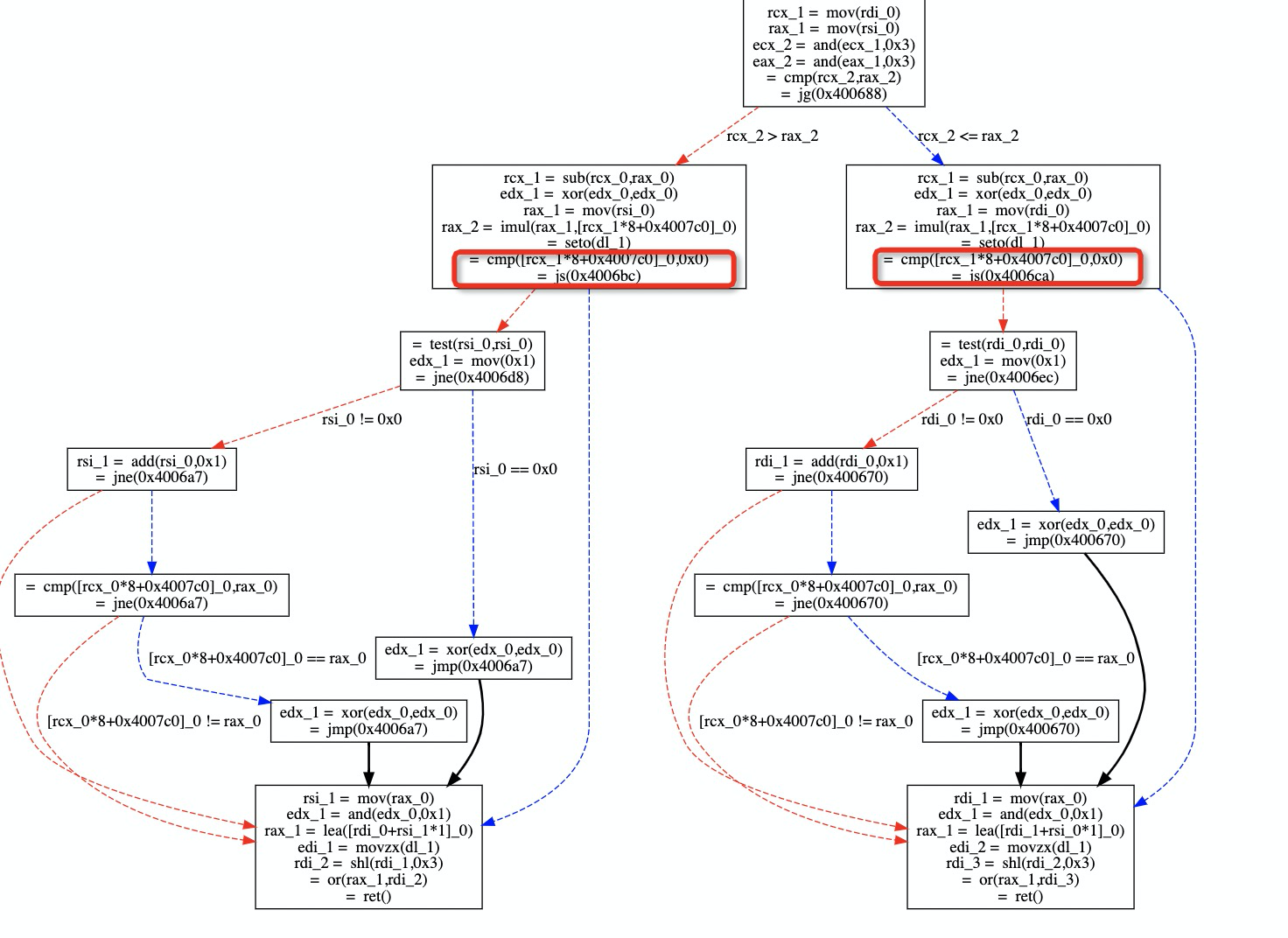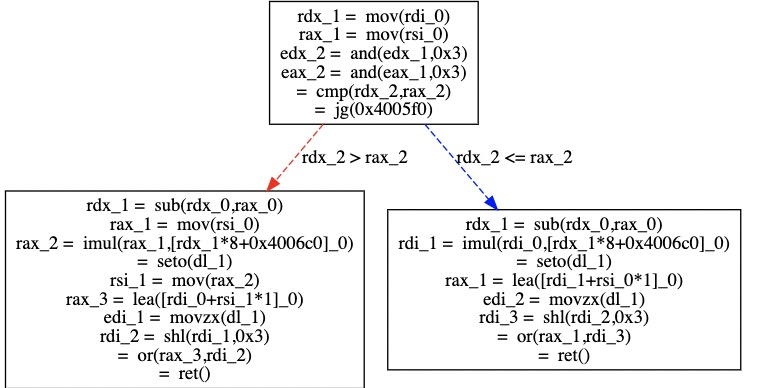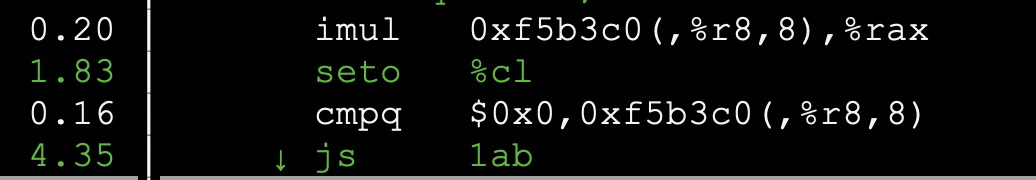# 指令级优化参考手册: 三

Posted by w@hidva.com on January 16, 2020

## 尽量避免有符号无符号整数的混用

constexpr uint64_t kM[] {
1,
};
int64_t X(int64_t l, int64_t r) {
int64_t ls = l & 0x3;
int64_t rs = r & 0x3;
int64_t dsts;
bool of;
if (ls > rs) {
dsts = ls;
of = __builtin_mul_overflow(r, kM[ls - rs], &r);
} else {
dsts = rs;
of = __builtin_mul_overflow(l, kM[ls - rs], &l);
}
l += r;
l |= (((int64_t)of) << 3);
return l;
}## 总是使用 int 及以上来作为整数类型

arithmetic operators do not accept types smaller than int as arguments, and integral promotions are automatically applied after lvalue-to-rvalue conversion, if applicable.

## 返回多个值时返回 struct 可能会更高效

struct X {
long a;
long b;
};

X f(X a1, X a2);


GCC 将使用 rdi 来传递 a1.a, rsi 来传递 a1.b, 通过 rdx, rcx 来传递 a2.a, a2.b, 通过 rax, rdx 来返回返回值对应的 a, b 字段信息. 不会涉及到对栈的访问.That means that the instruction pointer stored in each sample designates the place where the program was interrupted to process the PMU interrupt, not the place where the counter actually overflows. For this reason, care must be taken when interpreting profiles.